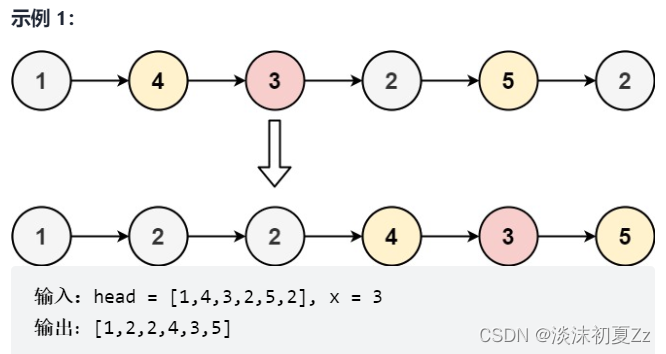﻿ Java详细讲解分析双指针法的使用_java_脚本之家
java# Java详细讲解分析双指针法的使用

## 1.判断链表是否有环```public class Solution {
//快慢指针法
while(fast != null && fast.next != null){
fast = fast.next.next;
low = low.next;
//此时相遇了
if(fast == low){
return true;
}
}
return false;
}
}
```

## 2.查找链表中间的元素```  //快慢指针法
while(fast != null && fast.next != null){
//慢指针走一步
low = low.next;
//快指针走两步
fast = fast.next.next;
}
//奇数，fast.next = null时，low即为所求
//偶数，fsat == null时，low即为所求
return low;
}
```

## 3.奇偶排序前奇后偶``` public ListNode oddEvenList(ListNode head) {
}
while(fastTail != null && fastTail.next != null){
//更新奇数节点时，奇数节点的后一个节点需要指向偶数节点的后一个节点
lowTail.next = fastTail.next;
lowTail = lowTail.next;
// 更新偶数节点时，偶数节点的后一个节点需要指向奇数节点的后一个节点
fastTail.next = lowTail.next;
fastTail = fastTail.next;
}
//合并两个链表
}
```

## 4.删除排序链表的重复元素``` public ListNode deleteDuplicates(ListNode head) {
//虚拟头节点法
ListNode cur = prev.next;
while(cur != null){
//引入next指针
ListNode next = cur.next;
if(next == null){
//只有一个元素
}
if(cur.val != next.val){
//cur不是重复节点，三指针都移动
cur = cur.next;
prev = prev.next;
}else{
//让next指针一直向后移动，直到与cur.val不相等的节点位置
while(next != null && cur.val == next.val){
next = next.next;
}
// 此时next指向了第一个不重复的元素
// 此时prev - next之间所有重复元素全部删除
prev.next = next;
cur = next;
}
}
}
```

## 5.三数之和``` public List<List<Integer>> threeSum(int[] nums) {
int n = nums.length;
Arrays.sort(nums);
List<List<Integer>> ans = new ArrayList<List<Integer>>();
// 枚举 a
for (int first = 0; first < n; ++first) {
// 需要和上一次枚举的数不相同
if (first > 0 && nums[first] == nums[first - 1]) {
continue;
}
// c 对应的指针初始指向数组的最右端
int third = n - 1;
int target = -nums[first];
// 枚举 b
for (int second = first + 1; second < n; ++second) {
// 需要和上一次枚举的数不相同
if (second > first + 1 && nums[second] == nums[second - 1]) {
continue;
}
// 需要保证 b 的指针在 c 的指针的左侧
while (second < third && nums[second] + nums[third] > target) {
--third;
}
// 如果指针重合，随着 b 后续的增加
// 就不会有满足 a+b+c=0 并且 b<c 的 c 了，可以退出循环
if (second == third) {
break;
}
if (nums[second] + nums[third] == target) {
List<Integer> list = new ArrayList<Integer>();
}
}
}
return ans;
}
```

## 6.分割链表``` public ListNode partition(ListNode head, int x) {
// 创建small和big两个小链表的头节点
// 按照升序插入，因此需要尾插
// 分别指向两个子链表的尾部
//遍历原链表，根据值放入small和big链表中
}else{
}
}
bigTail.next = null;
//拼接小链表和大链表
}
```

## 7.合并两个有序的数组```public void merge(int[] nums1, int m, int[] nums2, int n) {
int p1 = 0, p2 = 0;
int[] sorted = new int[m + n];
int cur;
while (p1 < m || p2 < n) {
if (p1 == m) {
cur = nums2[p2++];
} else if (p2 == n) {
cur = nums1[p1++];
} else if (nums1[p1] < nums2[p2]) {
cur = nums1[p1++];
} else {
cur = nums2[p2++];
}
sorted[p1 + p2 - 1] = cur;
}
for (int i = 0; i != m + n; ++i) {
nums1[i] = sorted[i];
}
}
```

## 8.两数之和—输入有序数组``` public int[] twoSum(int[] numbers, int target) {
int low = 0, high = numbers.length - 1;
while (low < high) {
int sum = numbers[low] + numbers[high];
if (sum == target) {
return new int[]{low + 1, high + 1};
} else if (sum < target) {
++low;
} else {
--high;
}
}
return new int[]{-1, -1};
}
```

## 9.长度最小的子数组

（力扣209）给定一个含有 n 个正整数的数组和一个正整数 target 。```//滑动窗口法
public int minSubArrayLen(int s, int[] nums) {
int n = nums.length;
if (n == 0) {
return 0;
}
int ans = Integer.MAX_VALUE;
int start = 0, end = 0;
int sum = 0;
while (end < n) {
sum += nums[end];
while (sum >= s) {
ans = Math.min(ans, end - start + 1);
sum -= nums[start];
start++;
}
end++;
}
return ans == Integer.MAX_VALUE ? 0 : ans;
}
```

## 10.排序链表1，分割 cut 环节： 找到当前链表中点，并从中点将链表断开（以便在下次递归 cut 时，链表片段拥有正确边界）； 我们使用 fast,slow 快慢双指针法，奇数个节点找到中点，偶数个节点找到中心左边的节点。 找到中点 slow 后，执行 slow.next = None 将链表切断。 递归分割时，输入当前链表左端点 head 和中心节点 slow 的下一个节点 tmp(因为链表是从 slow 切断的)。 cut 递归终止条件： 当head.next == None时，说明只有一个节点了，直接返回此节点。

2，合并 merge 环节： 将两个排序链表合并，转化为一个排序链表。 双指针法合并，建立辅助ListNode h 作为头部。 设置两指针 left, right 分别指向两链表头部，比较两指针处节点值大小，由小到大加入合并链表头部，指针交替前进，直至添加完两个链表。 返回辅助ListNode h 作为头部的下个节点 h.next。

```public ListNode sortList(ListNode head) {
while (fast != null && fast.next != null) {
slow = slow.next;
fast = fast.next.next;
}
ListNode tmp = slow.next;
slow.next = null;
ListNode right = sortList(tmp);
ListNode h = new ListNode(0);
ListNode res = h;
while (left != null && right != null) {
if (left.val < right.val) {
h.next = left;
left = left.next;
} else {
h.next = right;
right = right.next;
}
h = h.next;
}
h.next = left != null ? left : right;
return res.next;
}
```